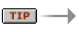# Number Sets, Infinity, and Zero Homework

Problem H1
Divide the number 1 by the numbers 1 through 10 consecutively. What conjectures can you make about rational numbers when represented as decimals?

Problem H2
If we think of division as a repeated subtraction, can you explain why it is impossible to divide by 0?

Problem H3
In a hotel with an infinite number of rooms and a counting number assigned to each, there is a “No Vacancy” sign outside. A traveler comes in and asks for a room for the night. How does the staff accommodate this traveler?Think of the traveler as one more element to add to a countably infinite set. Is the new set also countably infinite?

Problem H4
In a hotel with an infinite number of rooms and a counting number assigned to each, there is a “No Vacancy” sign outside. An infinite marching band — one where each member has a unique number on his or her uniform — enters and asks for a room for the night for each musician. How does the hotel staff accommodate everyone?Think of the band and the rooms as two infinite sets to be added together. What kind of set do you get? How can you put this new set into one-to-one correspondence with the counting numbers?

Problem H5
There’s an infinite chain of infinite hotels, each with a unique address on the street. All of them are full. But one night, very suddenly, all but one of them go out of business! How does the one remaining hotel accommodate all the stranded guests?Think of this as adding together an infinite number of infinite sets. This will be similar to putting rational numbers into one-to-one correspondence with the counting numbers.

Read History and Transfinite Numbers: Counting Infinite Sets.

History and Transfinite Numbers: Counting Infinite Sets.

### Solutions

Problem H1
1/1 = 1
1/2 = 0.5
1/3 = 0.333…
1/4 = 0.25
1/5 = 0.2
1/7 = 0.142857142857…
1/8 = 0.125
1/9 = 0.111…
1/10 = 0.1

Some rational numbers when expressed in decimal form will terminate, such as 0.5 or 0.125. Others will have repeating, non-terminating decimals where the repeating part can be a single digit, such as in 1/3 = 0.333…, or six digits, such as in 1/7 = 0.142857…

To further explore why this happens and why some rational numbers terminate and others don’t, go to Number and Operations, Session 7.

Problem H2
If we think of division as repeated subtraction, in essence, we are subtracting groups of 0 from the number we are dividing. It is easy to see that you could subtract groups of 0 infinitely many times and never exhaust the number you started with. Therefore, dividing by 0 is not defined.

Problem H3
The easiest solution would be to move everyone in the hotel one room over. This way, the first room would be freed up for the traveler. Notice that here you’ve added one element to an infinitely countable set. The result is still an infinitely countable set:Problem H4
To put everyone into a room, you would alternate the guests (G) already there and the marching band guests (MB). In other words, the guests who were already in the hotel would be moved into rooms with odd numbers:By combining the two infinite sets in this way, you still get a countably infinite set that can be put into one-to-one correspondence with the counting numbers.

Problem H5
By writing down all the rooms in all the hotels in an infinite two-dimensional matrix (see below), they can all then be put into one-to-one correspondence with the counting numbers, each of which corresponds to a particular room:Notice that here you have multiples of infinitely countable sets combined into one set. The new set is also countably infinite, which you’ve shown by putting it into one-to-one correspondence with the counting numbers.